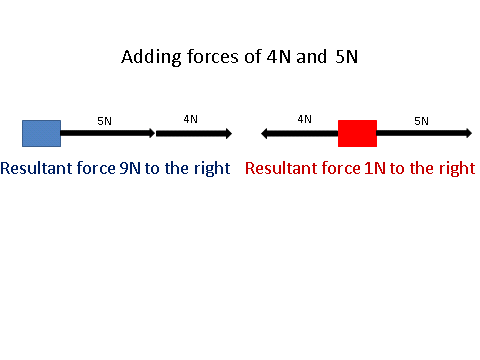# Forces

## Non Contact Forces

A force can change the__ shape__ or the __velocity __of an object. Forces are measured in Newtons (N).

There are two different types of forces: Contact and non-contact forces.

A non-contact force occurs when two objects are physically separated. Common examples of non-contact forces are:

• Gravitational
• Electrostatic
• Magnetic

## Contact Forces

A contact force occurs when two objects are__ physically touching__. Common examples of contact forces are:

• Friction
• Air resistance
• Tension
• Normal contact forces

As mentioned previously, all forces are __vector __quantities.

Since this is the case, we use arrows to show how large the force is and which direction it is acting in.

There are cases in which forces are acting in different directions.Since forces are vectors, you can add them up or subtract them from each other. You can only do this for forces that are acting on the same plane, so vertically or horizontally.

The overall force is called the resultant force. In the case of the blue box, the resultant force is 9N to the right and the red box is 1N to the right, since 5 - 4 = 1.

What is the resultant force on an object when it has a force of 12N acting upwards and 10N acting downwards?
Your answer should include: 2N / upwards
Give an example of a non-contact force
Your answer should include: gravitational / magnetic / electrostatic
Explanation: Gravitational, magnetic, electrostatic.
What is the unit of Force?
Your answer should include: Newton / Newtons / N